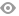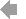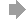Basic HTML VersionView Full Version
Page 10 - Catalogo completo Autolift
P. 10

``````9

ALZACRISTALLI ELETTRICI EQUIVALENTI DIFFERENT CODES, EQUAL WINDOW REGULATORS

ALFA ROMEO
AR 29 = FA 04

AUDI
AU 24SM = SA 24SM
CN 47 = PT 46 = TA 47                                        CN 21 = PT 14
CITROEN
CN 45 = PT 45 = TA 45                                 CN 28 = PT 32

CN 57 = FA 57 = PT 57                         CN 19 = FA 39 = PT 21          CN 20 = FA 43 = PT 27 = LC 24      CN 69 = FA 69 = PT 69

CN 76 = FA 76 = PT 76

DAEWOO
DW 02 = OV 06

DAF
DF 9080 = RT 6020 = VL 8050          DF 9090 = RT 6030 = VL 8060
FIAT

FA 71SM =LC 27SM       FA 04 = AR 29                FA 74SM = LC 29SM       FA 75SM = LC30SM

FA 57 = CN 57 = PT 57                         FA 57SM = CN 57SM = PT 57SM          FA 39 = CN 19 = PT 21    FA 43 = CN 20 = LC 24 = PT 27

FA 69 = CN 69 = PT 69  FA 69SCM = CN 69SCM = PT69SCM               FA 69SM = CN 69SM = PT69SM

FORD
FD 44 = SA 11 = VN 37          FD 44SCM = SA 11SCM = VN 37SCM                      FD 69SCM = SA 29SCM = VN 79SCM         FD 45 = SA 12 = VN 38

FD 45SCM = SA 12SCM = VN 38SCM FD 70SCM = SA30SCM = VN80SCM

HONDA
HN 31 = RR 31

HYUNDAI
HD 20 = MT 01

ISUZU
OV 27 = IS 06                OV 30 = IS 05
IVECO
FA 67 = NS 05 = OV 40 = RT 40                 FA 67SCM = NS 05SCM = OV 40SCM = RT 40SCM               FA 67SM = NS 05SM = OV 40SM = RT 40SM

LANCIA
LC 27SM = FA 71SM                             LC 29SM = FA 74SM              LC 30SM = FA 75SM        LC 24 = FA 43 = CN 20 = PT 27
MERCEDES
MR 17 = MR 23          MR 52 = VN 53      MR 52SM = VN 53SM
MITSUBISHI
MT 01 = HD 20
NS 67 = OV 67 = RT 67
NISSAN
NS 32 = FD 10          NS 44 = RT 33  NS 45 = OV 41 = RT 41

NS 05 = OV 40 = RT 40 = FA 67  NS 05SCM = OV 40SCM = RT 40SCM = FA 67SCM NS 05SM = OV 40SM = RT 40SM = FA 67SM                NS 67SM = OV 67SM = RT 67SM

OPEL
OV 27 = IS 06          OV 30 = IS 05          OV 50 = SK 18 (2000)          OV 06 = DW 02

OV 41 = RT 41 = NS 45 OV 41 = RT 40 = NS 05 = FA 67 OV 40SCM = RT 40SCM = NS 05SCM = FA 67SCM OV 40SM = RT 40SM = NS 05SM = FA 67SM

OV 67 = NS 67 = RT 67         OV 67SM = NS 67SM = RT 67SM
PEUGEOT
PT 46 = CN 47 = TA 47  PT 45 = CN 45 = TA 75                 PT 14 = CN 21 PT 32 = CN 28

PT 57 = CN 57 = FA 57                         PT 21 = CN 19 = FA 39          PT 27 = CN 20 = FA 43 = LC 24      PT 69 = CN 69 = FA 69

PT 76 = CN 76 = FA 76

PORSCHE
PS 04SM = VN 81SM            PS 05SM = VN 82SM
RENAULT
RT 33 = NS 44 RT 40 = NS 05 = OV 40 = FA 67 RT 40SCM = NS 05SCM = OV 40SCM = FA 67SCM RT 40SM = NS 05SM = OV 40SM = FA 67SM

RT 67 = NS 67 = OV 67          RT 67SM = NS 67SM = OV 67SM                   RT 6020 = DF 9080 = VL 8050        RT 6030 = DF 9090 = VL 8060
ROVER
RR 13 = HN 24                RR 31 = HN 31
SEAT
SA 14 = VN 46          SA 14SCM = VN 46SCM    SA 14SM = VN 46SM             SA 25SM = SA 15SM

SA 24SM = AU 24SM              SA 11 = FD 40 = VN 37                 SA 11SCM = FD 44SCM = VN 37SCM         SA 29SCM = FD 69SCM = VN 79SCM

SA 12SCM = FD 455SCM = VN 38SCM                     SA 30SCM = FD 70SCM = VN80SCM
SUBARU
SR 14 = SK 03                SR 15 = SK 04
TOYOTA
TA 47 = CN 47 = PT 46        TA 75 = CN 45 = PT 45
VOLKSWAGEN
VN 46 = SA 14          VN 46SCM = SA 14SCM    VN 46SM = SA 14SM             VN 72SM = VN 70SM

VN 37 = FD 44 = SA 11 VN 37SCM = FD 44SCM = SA 11SCM VN 79SCM = FD 69SCM = SA 29SCM VN 80SCM = FD 70SCM = SA 30SCM

VN 38 = FD 45 = SA 12 VN 38SCM = FD 45SCM = SA 12SCM VN 36 = SA 36 = SD 36 VN 81SM = PS 04SM

VN 82SM = PS 05SM      VN 53 = MR 52          VN 53SM = MR 52SM

VOLVO
VL 8050 = DF 9080 = RT 6020 VL 8060 = DF 9090 = RT 6030``````5   6   7   8   9   10   11   12   13   14   15